# AP Calculus BC Practice Test 44

### Test Information8 questions24 minutes

Calculator Allowed

1. Find the values of a and b that assure that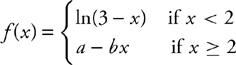is differentiable at x = 2.

2. The table shows some of the values of differentiable functions f and g and their derivatives. If h(x) = f(g(x)), then h′(2) equals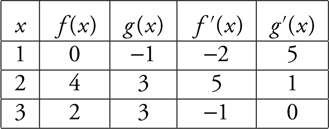3. Line l is tangent to the graph of a function f at the point (0, 1). If f is twice differentiable with f′ (0) = 2 and f″ (0) = 3. What is the approximate value of f (0.1) using line l?

4. Let f(x) be a differentiable function on the closed interval [1, 3]. The average value of f′ (x) on [1, 3] is

5. The position of a particle moving in the xy-plane at any time t is given as x(t) = 2 cos (4t) and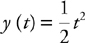. What is the speed of the particle at t = 1?

6.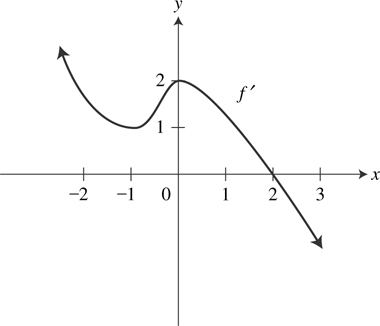The graph of f′ is shown above. Which of the following statements is/are true?

I. The function f is decreasing on the interval (-∞, -1).

II. The function f has an absolute maximum at x = 2.

III. The function f has a point of inflection at x = -1.

7. .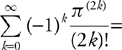8. The slope of the normal line to y = e-2x when x = 1.158 is approximately